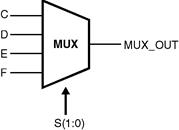Home > VHDL > Sequential Statements > Case statements

Case Statement :

Case statement has large sequences of the statements. CASE statement is used for sequential code along with IF, LOOP, and WAIT statements. The CASE statement is similar to WHEN statement. In CASE statement all the combinations are tested hence the keyword OTHERS is used. Another important keyword is NULL, which is used when no action take place. e.g. WHEN OTHERS =>NULL. At the backend of the tool case statement solves expression and compare it with choice value. The syntax is :

case expression is

when choices =>

{ sequential_statement }

{ when choices =>

{ sequential_statement } }

end case;

Ã˜ Example 1 :

WHEN "001" => decode <= value1;

WHEN "111" => decode <= value2;

WHEN "010" => decode <= value3;

WHEN "101" => decode <= value4;

WHEN OTHERS => decode <= value5;

END CASE ;

Ã˜ Example 2 : 4: 1 Multiplexurelibrary IEEE;

use IEEE.std_logic_1164.all;

entity mux is

port (c, d, e, f : in std_logic;

s :in std_logic_vector(1 downto 0);

muxout : out std_logic);

end mux;

architecture my_mux of mux is

begin

process (s, c, d, e, f) begin

case s is

when "00" => muxout <= c;

when "01" => muxout <= d;

when "10" => muxout <= e;

when others => muxout <= f;

end case ;

end process mux1;

end my_mux;